# Stokes phenomenon

(diff) ← Older revision | Latest revision (diff) | Newer revision → (diff)

The property that a functionmay have different asymptotic expressions when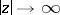in different domains of the complex-plane. G. Stokes demonstrated  that the solution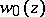of the so-called Airy equationwhich decreases for real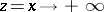, has the following asymptotic expansion when: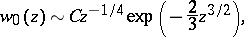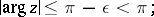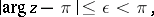where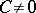is a constant. The functionis an entire function, while its asymptotic expansion is a discontinuous function.

The Stokes phenomenon also occurs for Laplace integrals, solutions of ordinary differential equations, etc. (see , ).

How to Cite This Entry:
Stokes phenomenon. Encyclopedia of Mathematics. URL: http://encyclopediaofmath.org/index.php?title=Stokes_phenomenon&oldid=12756
This article was adapted from an original article by M.V. Fedoryuk (originator), which appeared in Encyclopedia of Mathematics - ISBN 1402006098. See original article